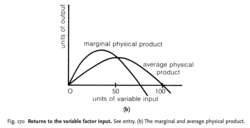# returns to the variable factor inputFig. 170 Returns to the variable factor input. See entry. (b) The marginal and average physical product.

## returns to the variable factor input

the rate of change of OUTPUT within the SHORT-RUN theory of supply, resulting from changes in the VARIABLE-FACTOR INPUT in a plant of a given (fixed) size. In the short run, some FACTOR INPUTS are variable but other inputs are fixed. A firm can change its rate of output only by combining more or fewer of the variable factors with the fixed factors: that is, by changing the proportions in which the factor inputs are used. As more and more of a variable input is used in conjunction with a given quantity of fixed input:
1. initially, as Fig. 170 (a) shows, there are increasing returns to the variable input: output increases more than proportionately to the increase in the variable input so the TOTAL PHYSICAL PRODUCT curve rises steadily and both MARGINAL PHYSICAL PRODUCT and AVERAGE PHYSICAL PRODUCT also increase;
2. CONSTANT RETURNS are then experienced, with the increase in output being exactly proportional to the increase in the variable input (marginal physical product and average physical product being constant);
3. DIMINISHING RETURNS to the variable input are then experienced (see Fig. 170) , with the increase in output being less than proportional to the increase in the variable input (the total physical product curve flattens out and both marginal physical product and average physical product decrease);
4. finally, negative returns may be experienced, with an increase in the variable input bringing about a fall in total output (the total physical product curve turns down and both marginal physical product and average physical product become negative). See ISOQUANT MAP.
Site: Follow: Share:
Open / Close